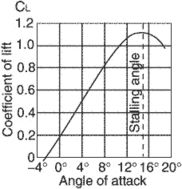# coefficient of lift

Also found in: Acronyms.

## coefficient of lift (CL)A form in which aerodynamic data is presented. A lift coefficient is dependent upon the angle of attack, shape of the wing section and plan form, condition of the wing surface, Mach number, and Reynolds number. Since most other factors are constant, CL values are plotted against the angle of attack. The value of CL rises up to the stalling angle, where it falls off rapidly. Lift coefficient (CL) = Lift (L)/Dynamic Pressure (q) × Wing Area (S) or CL = L/qS, or 2L/ρ V2S.
References in periodicals archive ?
Total Lift Force = Airplane Weight + Tail Down Force 2150 pounds = 2000 pounds + 150 pounds Table 1 Lift Force = Coefficient of Lift * Air Density / 2 * Wing Surface Area * [Airspeed.
l,pl] is the shape coefficient of lift, defined using the object's planform area.
The resulting yaw brings the left wing forward, decreasing [alpha] and thus bringing it back to the front side of the coefficient of lift curve and increasing lift.
Aircraft manufacturers, NASA and the de-ice equipment purveyors have done enough research to demonstrate even relatively benign ice accretion--if there is such a concept when it comes to icing--on a wing's leading can reduce the maximum coefficient of lift by as much as 30 percent, according to Dennis Newton's excellent treatise on the subject, Severe Weather Flying.

Site: Follow: Share:
Open / Close